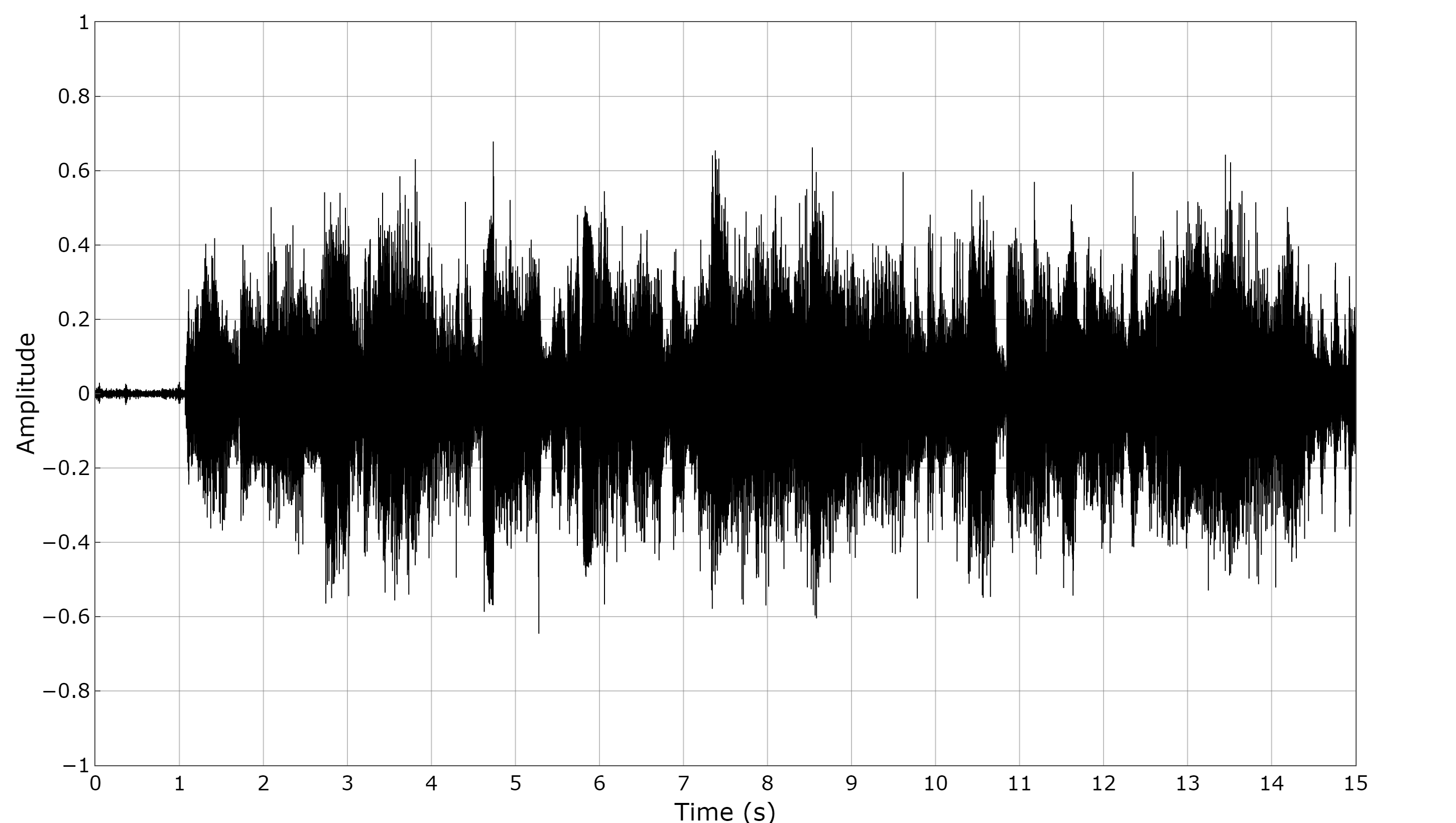# Time Domain PlotsBelow you will find examples for plotting:

• Short audio clips (~1s)
• Time domain plot short.r
• Audio 0.8s.wav
• Long audio clips (10s+)
• Time domain plot long.r
• Try your own WAV file!
• Vibration data (ms)
• Impulse plot.r
• Impulse data.txt

Example scripts and associated data files can be found here.

# Short Audio Clips (~1s)

We will use the package tuneR to read in a wav file and convert it to a series of amplitude data.

Read in the wav file using a dialog selection box:

```myFile <- file.choose()

Check the properties of the wav file using:

`str(sndObj)`

This will show you information such as the number of channels, sample rate, and bit depth.

Now we need to build a data frame that contains one column of time information and one of amplitude. First we’ll isolate the left channel:

`s1 <- sndObj@left`

Now we’ll convert the values from bit steps to values between -1 and 1:

`s1 <- s1 / 2^(sndObj@bit -1)`

Next we need to create a column of time values:

```filelength <- length(s1)
timeArray <- (0:(filelength-1)) / [email protected]
timeArray <- timeArray * 1000 #scale to milliseconds```

Now we can create the data frame and carry on as in the previous example.

`mydata <- data.frame(timeArray,s1)`# Long Audio Clips (10s+)

Due to the large number of samples that are being processed when creating a time domain plot the export time increases dramatically with file length. For example, a data set containing 1,000,000 samples ([email protected]) takes 1min to export. To reduce this time (at the expense of accuracy) we can throw some samples away:

`mydataextract <- mydata[c(TRUE,rep(FALSE,3)), ]`

This will extract the rows of our choosing. Using 3 will extract rows 1, 5, 10, 15 etc. Be careful not remove too many rows as this will make a very noticeable difference in your plots.Remember to remove line:

`timeArray <- timeArray * 1000 #scale to milliseconds`

As well as editing the yaxis formatting when plotting in seconds.

# Vibration Data (ms)

The plot below shows an impulse created from an impact hammer and the subsequent response from an accelerometer. The example data is provided in csv format.